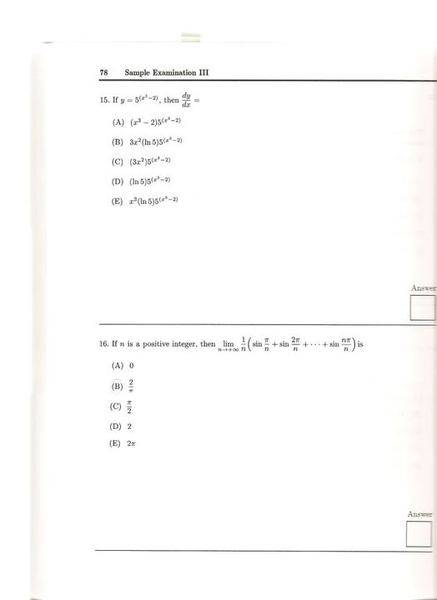# Limits & Riemman Sum

• lLovePhysics

## Homework Statement

Question regarding #16Riemman Sum

## The Attempt at a Solution

I know that the limit of the Riemman Sum is basically the integral. However, I do not know where to go from there. Do I need to use the Summation formulas? Thanks

can you write down the expression for a Riemann integral. something like:

$$\int_a^b f(x) dx = \lim_{N \to \infty} \sum_{n=1}^N ?$$

what goes in the question marks?

also, even though Riemann doesn't, assume things in the ? are equally spaced. that's usually good enough.

Last edited:
can you write down the expression for a Riemann integral. something like:

$$\int_a^b f(x) dx = \lim_{N \to \infty} \sum_{n=1}^N ?$$

what goes in the question marks?

also, even though Riemann doesn't, assume things in the ? are equally spaced. that's usually good enough.

Well, here's what I have so far:

$$\int_a^b f(x) dx = \lim_{N \to \infty} \sum_{n=1}^N (\frac{1}{N}\sin(\frac{\pi i}{N})$$

So what part of that sum is dx and what part is f(x)? After you know f(x), what is its integral?